# Class 10 Maths Important Questions for Polynomials

Given below are the polynomials class 10 important questions with answers
(a) Concepts questions
(b) Calculation problems
(c) Multiple choice questions(MCQ)
(e) True and False
(f) Match the column

## Match the column

A.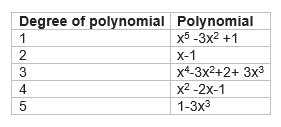B.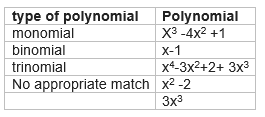C.
P(x)=5x3 -3x2+7x+2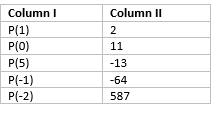D.
 Graph of polynomial Number of Zeros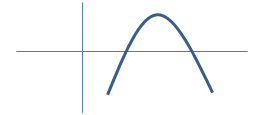012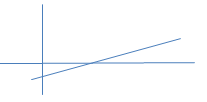3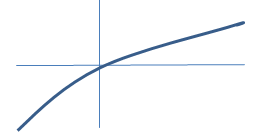4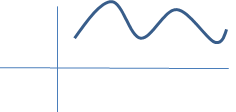5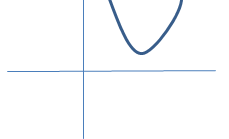6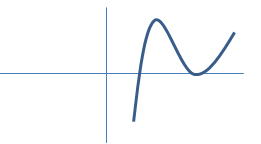7

E.
 Graph of polynomial Type of polynomial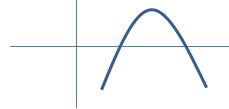Linear polynomialQuadratic polynomial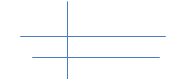Cubic polynomial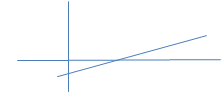Constant polynomial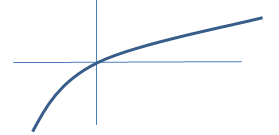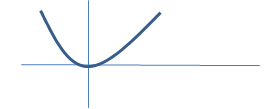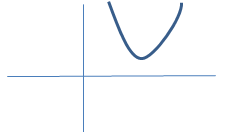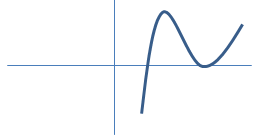## Multiple choice Questions

Question 1
Find the remainder  when x4+x3-2x2+x+1 is divided by x-1
a.1
b.5
c.2
d. 3

Question 2
Which of these identities is not true?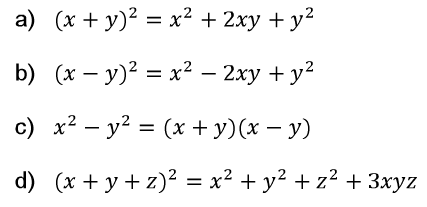Question 3
If a and b are the zeroes of the polynomial  x2-11x +30, Find the value of a3 + b3
a.134
b.412
c.256
d.341

Question 4
S(x) = px2+(p-2)x +2. If  2 is the zero of this polynomial,what is the value of p
a.-1
b.1/2
c. -1/2
d. +1

Question 5
if the zeroes of the quadratic equation are 11 and 2 ,what is expression for quadratic
a. x2-13x+22
b. x2-11x+22
c. x2-13x-22
d. x2+13x-22

Question 6
p(x) = x4 -6x3 +16x2 -25x +10
q(x) = x2-2x+k
It is given
p(x) = r(x) q(x) + (x+a)
Find the value of k and a
a.2,-2
b. 5 ,-5
c. 7,3
d. 3,-1

Question 7
A cubic polynomial is given below
S(x) =x3 -3x2+x+1
The zeroes of the polynomial are given as (p-q) ,p  and (p+q). What is the value p and q
a. p=1,q=√2 or -√2
b. p=1,q=2 or -2
c. p=1,q=1 or -1
d. None of these

Question 8
If the zeroes of the quadratic polynomial ax2 + bx + c, c ≠ 0 are equal, then
a. c and a have opposite signs
b. c and b have opposite signs
c. c and a have the same sign
d. c and b have the same sign

Question 9
What are the zeroes of the Polynomial $p(x)=6x^2 - 7x -3$
a. (3/2) , (-1/3)
b. (3/2) , (1/3)
c. (-3/2) , (1/3)
d. (-3/2) , (-1/3)

Question 10
What are the zeroes of the Polynomial $p(x)=x^2 + 7x +10$
a. (2) , (-5)
b. (-2) , (5)
c. (2) , (5)
d. (-2) , (-5)

## True or False statement

1. P(x) =x-1 and g(x) =x2-2x +1 .  p(x) is a factor of g(x)
2. The factor of 3x2 -x-4 are  (x+1)(3x-4)
3. Every linear polynomial has only one zero
4. Every real number is the zero’s of zero polynomial
5. A binomial may have degree 6
6. 1,2 are the zeroes of x2-3x+2
7. The degree of zero polynomial is not defined
8.  Graph of polynomial (x2-1) meets the  x-axis at one point
9. Graph of constant polynomial never meets x axis

## Factorize following

1. x2 +9x+18
2. 3x3 -x2-3x+1
3. x3-23x2+142x-120
4. 1+8x3

## Division of polynomial

s(x) =r(x) s(x) + w(x)
Find the value of r(x) and w(x) in each case
a. p(x) =x4+x3+2x2+3x+4
s(x) =x+2
b. p(x) =x4+4
s(x)=x2+x+1

## More Practice Questions

Question 1.
If the polynomial f(x) = x4 -6x3 + 16x2 - 25x + 10 is divided by another polynomial x2 -2x + k, the remainder comes out to be x + a, find k and a.

Question 2.
Find all the zeroes of the polynomial x4 - 3x3 + 6x - 4, if two of its zeroes are √2  and -√2

Question 3.
If p and q are he zeroes of the quadratic polynomial f(x) = x2 - 2x + 3, find a polynomial whose roots are:
1. p + 2, q + 2
2. (p-1)/(p+1) , (q-1)/(q+1)
Question 4.
For what value of k, -7 is the zero of the polynomial 2x2 + 11x + (6k - 3)? Also find the other zero of the polynomial

Question 5.
What must be added to f(x) = 4x4 + 2x3 - 2x2 + x - 1 so that the resulting polynomial is divisible by g(x) = x2 + 2x -3?

Question 6.
Find k so that x2 + 2x + k is a factor of 2x4 + x3 - 14 x2 + 5x + 6. Also find all the zeroes of the two polynomials.
Question 8.
Find the zeroes of 2x3 - 11x2 + 17x - 6.
Question 9.
If (x - 2) and [x - ½ ] are the factors of the polynomials qx2 + 5x + r prove that q = r

Question 10.
Find k so that the polynomial x2 + 2x + k is a factor of polynomial 2x4 + x3 - 14x2 + 5x + 6. Also, find all the zeroes of the two polynomials.

Question 11.
On dividing p(x) = x3 - 3x2 + x + 2 by a polynomial q(x), the quotient and remainder were x - 2 and -2x + 4, respectively. Find g(x).

Question 12.
a, b, c are zeroes of cubic polynomial x3 - 2x2 + qx - r. If a + b = 0 then show that 2q = r.
Question 13.
Find the remainder when x51 +51 is divided by (x+1).
Question 14.
a,b and c are zeroes of polynomial x3 + px2 + qx + 2 such that a b + 1 = 0. Find the value of 2p + q + 5.

Question 15.
Find the quadratic polynomial, the sum and product of whose zeroes are 4 and 1, respectively

Go back to Class 10 Main Page using below links

### Practice Question

Question 1 What is $1 - \sqrt {3}$ ?
A) Non terminating repeating
B) Non terminating non repeating
C) Terminating
D) None of the above
Question 2 The volume of the largest right circular cone that can be cut out from a cube of edge 4.2 cm is?
A) 19.4 cm3
B) 12 cm3
C) 78.6 cm3
D) 58.2 cm3
Question 3 The sum of the first three terms of an AP is 33. If the product of the first and the third term exceeds the second term by 29, the AP is ?
A) 2 ,21,11
B) 1,10,19
C) -1 ,8,17
D) 2 ,11,20